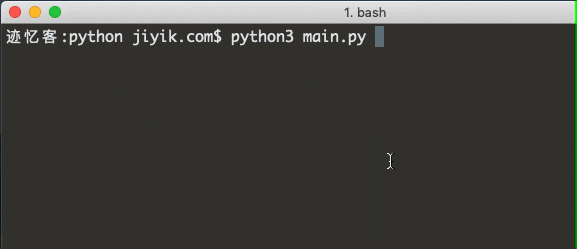# 迹忆客专注技术分享

## Python 中检查输入是否是为列表

``````import ast

# ✅ Check if variable stores a list
my_list = ['a', 'b', 'c']

if isinstance(my_list, list):
print('The variable stores a list')

print(type(my_list))  # 👉️ <class 'list'>
# ------------------------------------------

# ✅ Check if user input is list (e.g. ['a', 'b',])
try:
input_list = ast.literal_eval(
input('Enter a valid Python list, e.g. tasks=["walk the dog"]: ')
)
except ValueError:
print('The provided value is not a list')

print(input_list)  # 👉️ ['a', 'b']

# ------------------------------------------

# ✅ Split value from user input into a list

input_list = input('Enter multiple, space-separated values: ').split()

print(input_list)  # 👉️ ['hello' , 'world']
``````

``````my_list = ['a', 'b', 'c']

if isinstance(my_list, list):
print('The variable stores a list')

print(type(my_list))  # 👉️ <class 'list'>
``````

``````import ast

try:
input_list = ast.literal_eval(
input('Enter a valid Python list, e.g. ["walk the dog"]: ')
)
except ValueError:
print('The provided value is not a list')

print(input_list)  # 👉️ ['a', 'b']
```````ast.literal_eval` 方法允许我们安全地评估包含 Python 文字的字符串。

``````input_list = input('Enter multiple, space-separated values: ').split()

print(input_list)  # 👉️ ['hello' , 'world']
``````

`str.split()` 方法使用分隔符将字符串拆分为子字符串列表。

`str.split()` 方法在没有参数的情况下被调用时，它会将连续的空白字符视为单个分隔符。

``````input_list = input('Enter multiple, comma-separated values: ').split(',')

print(input_list)  # 👉️ ['hello' , 'world']
``````

`str.split()` 方法采用以下 2 个参数：

• separator 在每次出现分隔符时将字符串拆分为子字符串
• maxsplit 最多完成最大拆分（可选）## 热门标签